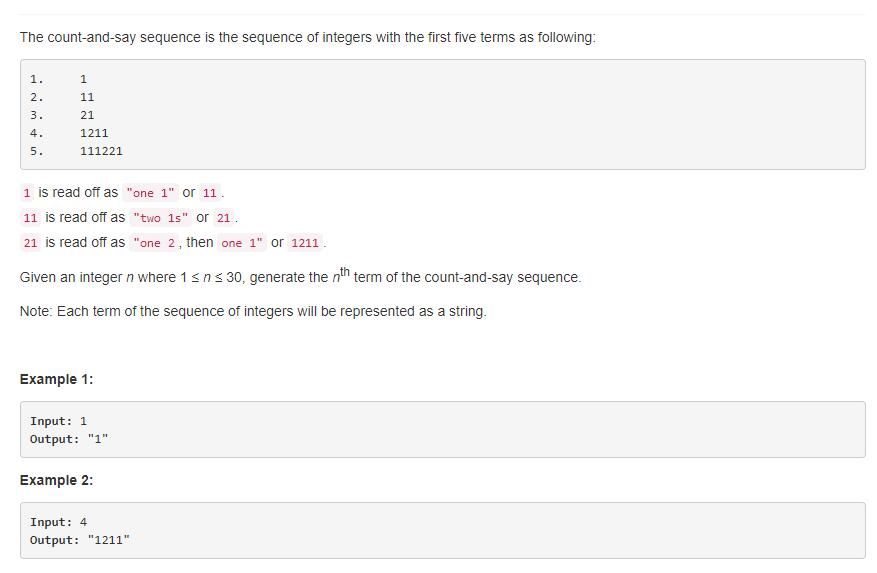题目描述（简单难度）解法一 递归

1 重复 3 次，就是 31，2 重复 2 次就是 22，1 重复 1 次 就是 11，所以最终结果就是 312211。

public String countAndSay(int n) {
//第一行就直接输出
if (n == 1) {
return "1";
}
//得到上一行的字符串
String last = countAndSay(n - 1);
//输出当前行的字符串
return getNextString(last);

}

private String getNextString(String last) {
//长度为 0 就返回空字符串
if (last.length() == 0) {
return "";
}
//得到第 1 个字符重复的次数
int num = getRepeatNum(last);
// 次数 + 当前字符 + 其余的字符串的情况
return num + "" + last.charAt(0) + getNextString(last.substring(num));
}

//得到字符 string 的重复个数，例如 "111221" 返回 3
private int getRepeatNum(String string) {
int count = 1;
char same = string.charAt(0);
for (int i = 1; i < string.length(); i++) {
if (same == string.charAt(i)) {
count++;
} else {
break;
}
}
return count;
}

解法二 迭代

public String countAndSay(int n) {
String res = "1";
//从第一行开始，一行一行产生
while (n > 1) {
String temp = "";
for (int i = 0; i < res.length(); i++) {
int num = getRepeatNum(res.substring(i));
temp = temp + num + "" + res.charAt(i);
//跳过重复的字符
i = i + num - 1;
}
n--;
//更新
res = temp;
}
return res;

}
//得到字符 string 的重复个数，例如 "111221" 返回 3
private int getRepeatNum(String string) {
int count = 1;
char same = string.charAt(0);
for (int i = 1; i < string.length(); i++) {
if (same == string.charAt(i)) {
count++;
} else {
break;
}
}
return count;
}